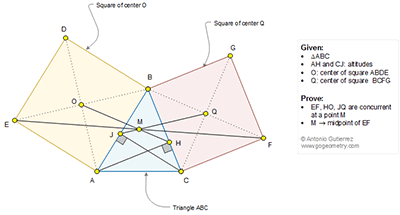## Thursday, December 8, 2016

### Geometry Problem 1293 Triangle, Altitudes, Two Squares, Center, Concurrent Lines, Midpoint

Geometry Problem. Post your solution in the comment box below.
Level: Mathematics Education, High School, Honors Geometry, College.

Details: Click on the figure below.1.Connect OA and OB
Observe that qua. OBHA is cyclic
So ∠BAH=∠BOH
In triangle ABH , ∠BAH complement to ∠B => ∠BOH complement to ∠B
So ∠BOH supplement to ∠OBF => OH //BF
Since O is the midpoint of EB and OH//BF=> OH cut EF at midpoint M
Similarly QJ also cut EF at midpoint M

2.Quad. AOBH is cyclic,Angle BOH= Angle BAH =90-B,Angle OBQ=90+B,Hence OH is parallel to BF. Since O is midpoint of BE, line OH will pass pass through midpoint of EF.
Similarly QJ is parallel to BE and passes through midpoint of EF. Hence QJ, OH and EF are concurrent at mid point of EF.

3.m(BHA) = m(BOA) = 90 => OABH is cyclic quadrilateral
since m(BAO) = 45 => m(BHO) = 45 => m(OHA) = 45 --------(1)
Extend AH to meet BF at P and consider the triangle BAP
we have
m(ABP) = B+45
m(BAP) = 90-B
=> m(BPA) = 45 ----(2)
From (1) and (2), we can say OH||BF
Since O is mid-point of BE, from mid-point theorem, M is the midpoint of EF
similarly QCJB can be found cyclic and thus JQ intersects EF at M